# XLOOKUP Function in Excel With Examples

• Difficulty Level : Expert
• Last Updated : 01 Jun, 2021

XLOOKUP Function helps us to search value in a horizontal or vertical dataset and return the relative value in some other row or column. In this article, we will look XLOOKUP Function in Excel.

Syntax:

`=XLOOKUP(lookup_value, lookup_array, return_array, [if_not_found], [match_mode],[search_mode])`

Parameter:

• lookup_value: The value which we want to search
• lookup_array: The range in which we want to search
• if_not_found (Optional): The text which we want to return if the value is not found
• match_mode (Optional): Here we can specify the type of match we want.
• search_mode (Optional): where we can specify how the function should look up the value.

Example 1: To get a Lookup Value

Follow the below steps to Lookup a value:

Step 1: Format your data.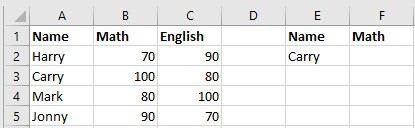Now, if we want to get the math marks of Carry then follow the next step

Step 2: We will enter =XLOOKUP(E2,A2:A5,B2:B5) in F2 cell.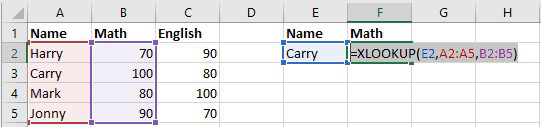Then we will get the math marks of Carry.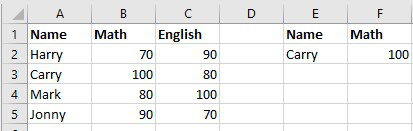Example 2: To Lookup and get the value of the entire row

Follow the below steps to lookup values of the entire row:

Step 1: Format your data.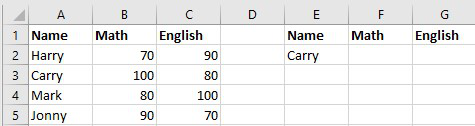Now, if we want to get the Math and English marks of Carry then follow next step

Step 2: We will enter =XLOOKUP(E2,A2:A5,B2:C5) in F2 cell.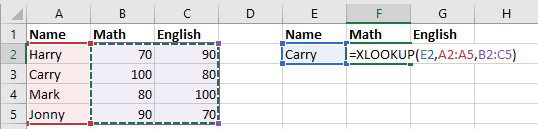Then we will get the Math and English marks.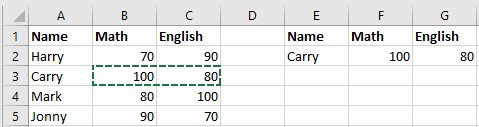Example 3: To get value using nested XLOOKUP function

Follow the below steps to use nested XLOOKUP functions:

Step 1: Format your data.Now, if we want to get the math marks of Carry by two-way lookup then follow the next step.

Step 2: We will enter =XLOOKUP(F1,B1:C1,XLOOKUP(E2,A2:A5,B2:C5)) in F2 cell.

Here, XLOOKUP(E2,A2:A5,B2:C5) is{100,80} which is a array mark of Carry. In the outer XLOOKUP formula, we are looking for the subject name which is in F1 cell and the lookup array is B1:C1.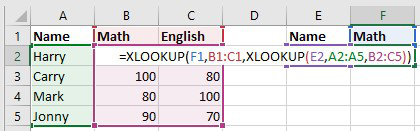Then we will get the math marks of carry.Example 4: When Lookup value is Not Found.

Follow the below steps when the LOOKUP function returns the Not Found message:

Step 1: Format your data.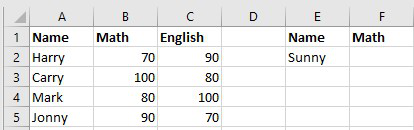If we want the marks of Sunny who is not in the dataset

Step 2: We will enter =XLOOKUP(E2,A2:A5,B2:B5,”Not Found”) in F2 cell.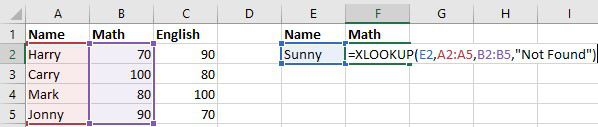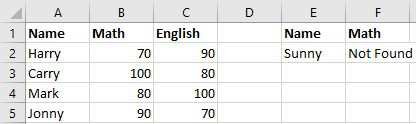Example 5: To find the sum of a range using the SUM function.

Follow the below steps to find the sum of a range:

Step 1: Format your data.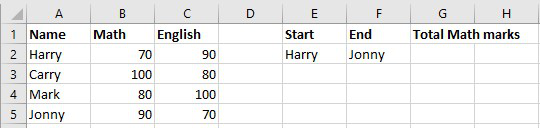If we want the total math marks from Harry to Jonny then do the next step

Step 2: We will enter =SUM(XLOOKUP(E2,A2:A5,B1:B6):XLOOKUP(F2,A2:A5,B1:B6)) in G2 cell this simply means =SUM(\$B\$2:\$B\$5).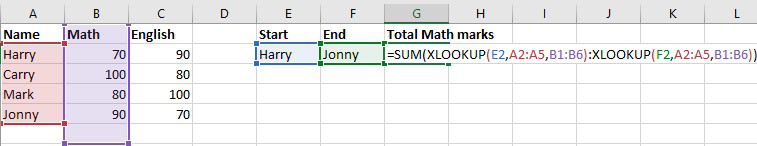Then we will get the sum.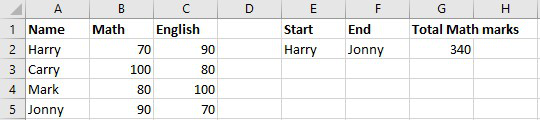Example 6: Horizontal Lookup

Step 1: Format your data.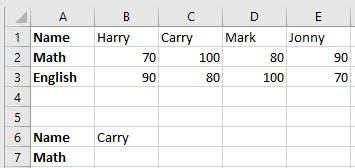Now, if we want to get the math marks of Carry then follow the next step

Step 2: We will enter =XLOOKUP(B6,B1:E1,B2:E2) in B7 cell.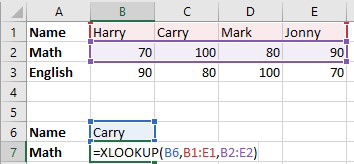Then we will get the math marks of Carry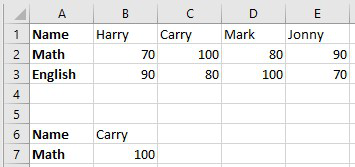My Personal Notes arrow_drop_up